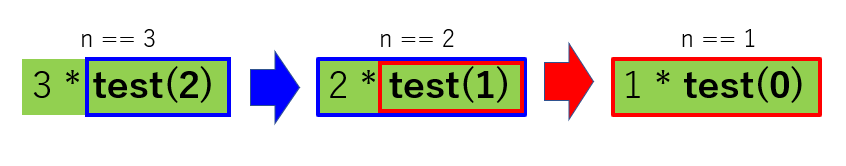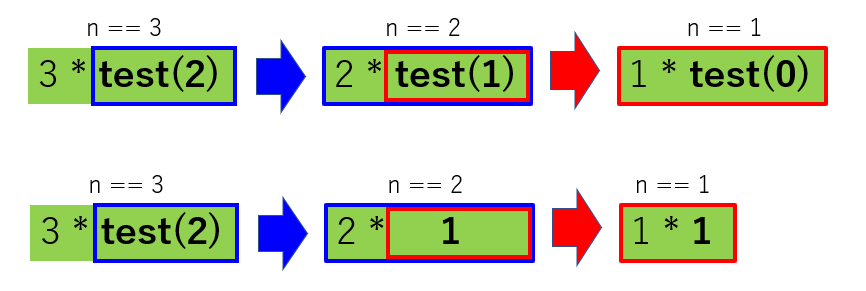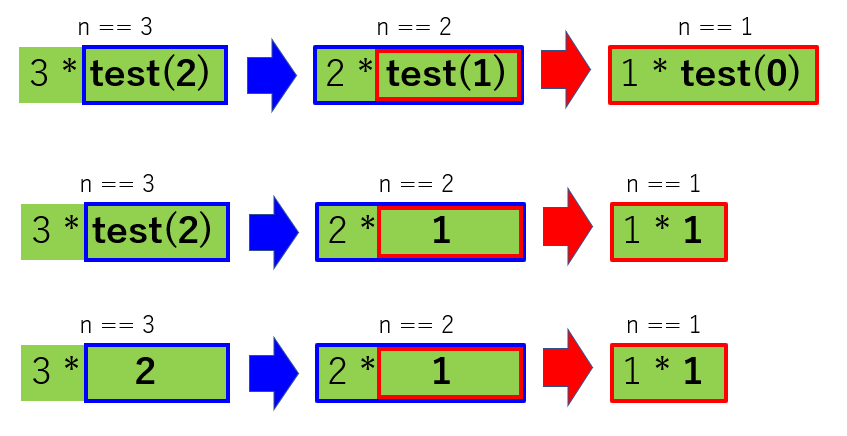# [PYTHON] Try to factorial with recursion

Good evening. I tried to be a beginner I hope it will be helpful to everyone.

For example, how can we achieve 3! (= 3 * 2 * 1)?

``````print(3*2*1)
``````

It's done (laughs)! Then, why not write it like this using def?

#### `test.py`

``````
def test(n:int=3):
if n>1:
return n * test(n-1)
else:
return 1

print(test())
``````

I hurriedly tried it with the initial value of 3. For the time being, let's substitute them one by one. First, when n == 3.

#### `test.py`

``````
# n ==When 3
def test(n:int=3):
#n ==Since it is 3, it goes inside if
if n>1:
#return 3 * test(3-1)
return n * test(n-1)
else:
return 1

print(test())
``````

Hooray. I can get out because the return has arrived !! 3 * test(2) !? You can't finish without knowing test (2). There is no way. I'm not sure, but consider n == 2, that is, test (2).

#### `test.py`

``````
# n ==When
def test(n:int=2):
#n ==2 so go inside if
if n>1:
#return 2 * test(2-1)
return n * test(n-1)
else:
return 1

print(test())
``````

Hmm !? return 2 * test (1)!? Test () appears again, but this time it is test (1). It can't be helped, let's try test (1) as well.

#### `test.py`

``````
# n ==When 1
def test(n:int=1):
if n>1:
return n * test(n-1)
#n ==Since it is 1, it goes inside else
else:
#Returns 1
return 1

print(test())
``````

For the time being, when n == 1, it is return 1, so I found that the answer is 1. I'm glad I finally met an integer (laughs)

Let's organize it once.After executing n == 3 as shown in the figure, n == 2 appeared, and then n == 1 appeared. As an image, 2 * test (1) is embedded in test (2), It seems that 1 * test (0) is embedded in test (1).

What we now know is that 1 * test (0) is 1 * 1, That is, it is just 1.

Alright, ignore 3 * test (2) once Let's substitute 1 * test (0) for test (1) of 2 * test (1) in the center of the figure below.Since 2 * test (1) == 2 * {1 * test (0)}, we know that 2 * 1 = 2. That means. .. ..3 * test (2) == 3 * {2 * test (1)} == 3 * {2 * 1}. With this, I managed to express 3 !.

As mentioned above, when a certain event includes itself, It is said to be recursive.

Using this idea, not only 3! But also the factorial of n can be expressed, of course. It's a simple description, but it's complicated when you think about it properly. It was interesting, thank you for your hard work (≧ ▽ ≦).